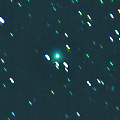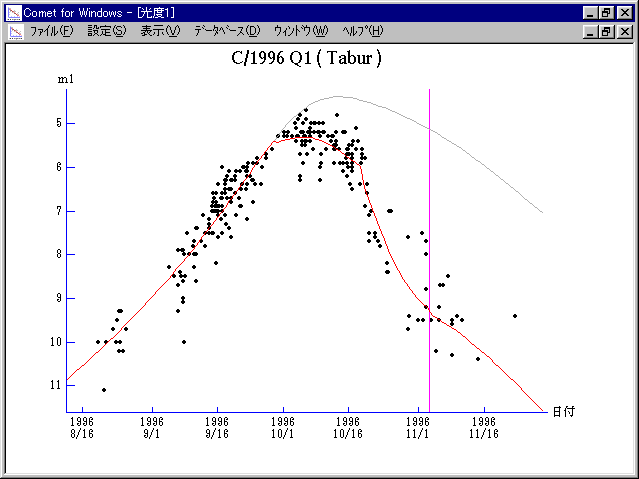# \$B%?%\$%P!

C/1996 Q1 ( Tabur )###\$B%W%m%U%#!<%k(B

 \$BH/8+F|(B 1996\$BG/(B8\$B7n(B19\$BF|(B \$BH/8+8wEY(B 10\$BEy(B \$BH/8+ Vello Tabur (Wanniassa, Australian Capital Territory)

###\$B###\$B50F;MWAG(B

```   The following improved orbital elements  by Kenji Muraoka, are
from 170 observations  1996 Aug. 21  to  Oct. 16, perturbations
by 9 Planets, Moon and 5 minor planets  were taken into account.
The mean residual is +/- 0.84 arc seconds.

Epoch  =  1996 Nov. 13.0  TT       JDT = 2450400.5
T  =  1996 Nov.  3.52909       +/- 0.00271 (m.e.) TT
Peri. =   57.40985                +/- 0.00239
Node  =   31.40097                +/- 0.00147   (2000.0)
Incl. =   73.35731                +/- 0.00147
q  =    0.8398150              +/- 0.0000137 AU
e  =    0.9987931              +/- 0.0001381
1/a  =   +0.0014371              +/- 0.0001644 1/AU
( P  =  18356 years )
orig. 1/a  =   +0.0019533
fut.  1/a  =   +0.0013944
```

###\$B@1?^(B1996\$BG/(B 5\$B7n(B 7\$BF|!A(B1996\$BG/(B 9\$B7n(B29\$BF|(B1996\$BG/(B 9\$B7n(B19\$BF|!A(B1996\$BG/(B11\$B7n(B 3\$BF|(B1996\$BG/(B10\$B7n(B 4\$BF|!A(B1997\$BG/(B 8\$B7n(B10\$BF|(B

###\$B8wEYJQ2=(B

```        m1 =  6.6 + 5 log\$B&\$(B + 15.0 log r  [   ,-35]  (              \$B!A(B1996\$BG/(B 9\$B7n(B29\$BF|(B)
m1 =  7.2 + 5 log\$B&\$(B -  3.5 log r  [-35,-15]  (1996\$BG/(B 9\$B7n(B29\$BF|!A(B1996\$BG/(B10\$B7n(B19\$BF|(B)
m1 =  3.5 + 5 log\$B&\$(B - 80   log r  [-15,  0]  (1996\$BG/(B10\$B7n(B19\$BF|!A(B1996\$BG/(B11\$B7n(B 3\$BF|(B)
m1 = 11.2 + 5 log\$B&\$(B + 20.0 log r  [  0,   ]  (1996\$BG/(B11\$B7n(B 3\$BF|!A(B              )

\$B"((B \$B3%?'\$N6J@~\$O!"(Bm1 = 6.6 + 5 log\$B&\$(B + 15.0 log r \$B\$N8wEY6J@~\$G\$9!#(B
```##### \$B50F;MWAG\$OB<2,7r<#;a\$N7W;;\$K\$h\$k\$b\$N\$G\$9!#(B \$B@1?^\$O(B StellaNavigator Ver.2.0 for Windows (\$B%"%9%H%m%"!<%D(B \$BJTCx(B / \$B%"%9%-!<=PHG6I4)(B) \$B\$G:n@.\$7\$?\$b\$N\$G\$9!#(B \$B8wEY%0%i%U\$O(BComet for Windows\$B\$G:n@.\$7\$?\$b\$N\$G\$9!#(B